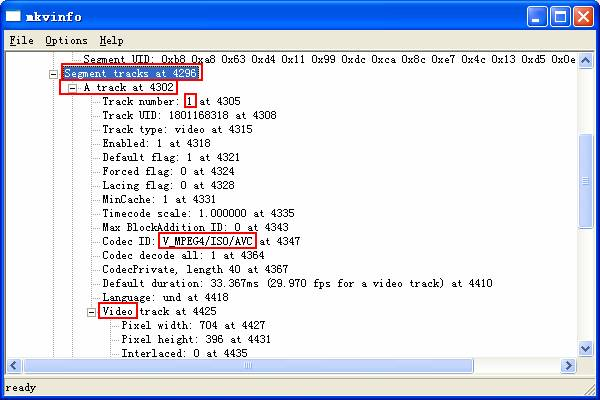﻿ 后期AVS教程(3) - 蜗牛的壳

Directshowsource("文件名",fps=帧速率) 使用类似Windows自带的媒体播放器打开文件的方式调用片源，效率远远低于AviSource。不到万不得已请不要使用。
Selectevery(n,n1,n2,n3……) 这个是Selectevery的一个新语法，意思是以n为周期，取每一个周期里的第n1 n2 n3……帧。这可能不太好理解，举例：

1 、片源的加载

DirectshowSource语句是加载视频的语句，与AVISource不同的是，Directshowsource使用Directshow方式加载视频（也就是WindowsMediaPlayer使用的那种加载方式）。使用Directshowsource语句加载视频最好要指定fps，比如fps=29.97。事实上，WindowsMediaPlayer配合各种Splitter（就是把封装格式解来播放用的，比如Windows自带AVISplitter）可以播放各种格式，甚至RMVB也可以。

2 、VFR的还原

[code]
b0=trim(0,40).selectevery(1,1,1,1,1,1).assumefps(119.88)
b1=trim(41,122).selectevery(1,1,1,1,1).assumefps(119.88)
b2=trim(123,412).selectevery(1,1,1,1,1,1).assumefps(119.88)
b3=trim(413,2624).selectevery(1,1,1,1,1).assumefps(119.88)
b4=trim(2625,2836).selectevery(1,1,1,1,1,1).assumefps(119.88)
b5=trim(2837,2926).selectevery(1,1,1,1,1).assumefps(119.88)
b6=trim(2927,3600).selectevery(1,1,1,1,1,1).assumefps(119.88)
b7=trim(3601,3840).selectevery(1,1,1,1,1).assumefps(119.88)
b8=trim(3841,8435).selectevery(1,1,1,1,1,1).assumefps(119.88)
b9=trim(8436,8547).selectevery(1,1,1,1,1).assumefps(119.88)
b10=trim(8548,13954).selectevery(1,1,1,1,1,1).assumefps(119.88)
b11=trim(13955,14034).selectevery(1,1,1,1,1).assumefps(119.88)
b12=trim(14035,14884).selectevery(1,1,1,1,1,1).assumefps(119.88)
b13=trim(14885,14950).selectevery(1,1,1,1,1).assumefps(119.88)
b14=trim(14951,15401).selectevery(1,1,1,1,1,1).assumefps(119.88)
b15=trim(15402,15781).selectevery(1,1,1,1,1).assumefps(119.88)
b16=trim(15782,17480).selectevery(1,1,1,1,1,1).assumefps(119.88)
b17=trim(17481,17720).selectevery(1,1,1,1,1).assumefps(119.88)
b18=trim(17721,32489).selectevery(1,1,1,1,1,1).assumefps(119.88)
b19=trim(32490,34286).selectevery(1,1,1,1,1).assumefps(119.88)
b20=trim(34287,34558).selectevery(1,1,1,1,1,1).assumefps(119.88)
b21=trim(34559,34632).selectevery(1,1,1,1,1).assumefps(119.88)
b22=trim(34633,35013).selectevery(1,1,1,1,1,1).assumefps(119.88)
b0+b1+b2+b3+b4+b5+b6+b7+b8+b9+b10+b11+b12+b13+b14+b15+b16+b17+b18+b19+b20+b21+b22
[/code]

3 、挂字幕

4 、去D帧

5 、压制

6 、封装

7 、附录：如何拆MKV

AVI的音频可以使用VDM来解，那么MKV里面的音频呢？